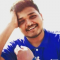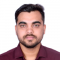## How to plot two dotted lines and set marker using Matplotlib?Updated on 16-Mar-2021 10:15:42

1K+ Views

In this program, we will plot two lines using the matplot library. Before starting to code, we need to first import the matplotlib library using the following command −Import matplotlib.pyplot as pltPyplot is a collection of command style functions that make matplotlib work like MATLAB.AlgorithmStep 1: Import matplotlib.pyplot Step 2: Define line1 and line2 points. Step 3: Plot the lines using the plot() function in pyplot. Step 4: Define the title, X-axis, Y-axis. Step 5: Display the plots using the show() function.Example Codeimport matplotlib.pyplot as plt line1_x = [10, 20, 30] line1_y = [20, 40, 10] line2_x = ... Read More

## Change figure window title in pylab(Python)Updated on 16-Mar-2021 10:07:24

2K+ Views

Using pylab.gcf(), we can create a fig variable and can set the fig.canvas.set_window_title('Setting up window title.') window title.StepsUsing gcf() method, get the current figure. If no current figure exists, a new one is created using ~.pyplot.figure().Set the title text of the window containing the figure, using set_window_title() method.. Note that this has no effect if there is no window (e.g., a PS backend).ExamplePlease use Ipython and follow the steps given below -In : from matplotlib import pylab In : fig = pylab.gcf() In : fig.canvas.set_window_title('Setting up window title.')OutputRead More

## Change x axes scale in matplotlibUpdated on 15-Mar-2021 08:44:15

12K+ Views

Using plt.xticks, we can change the X-axis scale.StepsUsing plt.plot() method, we can create a line with two lists that are passed in its argument.Add text to the axes. Add the text *s* to the axes at location *x*, *y* in data coordinates, using plt.text() method, where the font size can be customized by changing the font-size value.Using xticks method, get or set the current tick locations and labels of the X-axis.To show the figure, use plt.show() method.Exampleimport matplotlib.pyplot as plt plt.plot([1, 2, 4], [1, 2, 4]) plt.text(2, 3, "y=x", color='red', fontsize=20) plt.xticks([1, 2, 3, 4, 5])   ... Read More

## How to change fonts in matplotlib (python)?Updated on 15-Mar-2021 08:42:43

2K+ Views

Using plt.text() method, we can increase the font size.StepsUsing plt.plot() method, we can create a line with two lists that are passed in its argument.Add text to the axes. Add the text *s* to the axes at location *x*, *y* in data coordinates, using plt.text() method. Font size can be customized by changing the font-size value.To show the figure, use plt.show() method.Exampleimport matplotlib.pyplot as plt plt.plot([1, 2, 4], [1, 2, 4]) plt.text(2, 3, "y=x", color='red', fontsize=20) # Increase fontsize by increasing value. plt.show()Output

## Python matplotlib multiple barsUpdated on 15-Mar-2021 08:41:20

249 Views

We can use a user-defined method, autolabel, to annotate the axis value. Before that, we can initialize the fig and ax using plt.subplots() method.StepsCreate lists, labels, men_means, and women_means with different data elements.Return evenly spaced values within a given interval, using numpy.arrange() method.Set the width variable i.e., width=0.35.Create fig and ax variables using subplots method, where default nrows and ncols are 1.The bars are positioned at *x* with the given *align*\ment. Their dimensions are given by *height* and *width*. The vertical baseline is *bottom* (default 0), so create rect1 and rect2 using plt.bar() method.Set the Y-axis label using plt.ylabel() method.Set ... Read More

## Annotate bars with values on Pandas bar plots in PythonUpdated on 15-Mar-2021 08:39:08

249 Views

In this program, we can create a data frame and can plot a bar using df.plot.bar(x='lab', y='value', color='#5fba34') plot.StepsUsing Panda’s dataframe, we can create a data frame with the given dictionary, where the keys are lab and value, values of these keys are lists, respectively.Using Pandas plot.bar() method, we can create a vertical bar plot. A bar plot is a plot that presents categorical data with rectangular bars with lengths proportional to the values that they represent.To show the figure, use the plt.show() method.Exampleimport pandas as pd from matplotlib import pyplot as plt df = pd.DataFrame({'lab': ['A', 'B', 'C'], ... Read More

## Getting vertical gridlines to appear in line plot in matplotlibUpdated on 15-Mar-2021 08:37:50

2K+ Views

Using plt.grid(axis="x") method, we can plot vertical gridlines.StepsMake a list of numbers.Set the X-axis label using plt.xlabel() method.Set the Y-axis label using plt.ylabel() method.Toggle the gridlines, and optionally set the properties of the lines, using plt.grid() method.To show the figure, use the plt.show() method, where the argument axis can be “x”, “y” or “both”.Examplefrom matplotlib import pyplot as plt plt.plot([0, 5], [0, 5]) plt.ylabel("Y-axis ") plt.xlabel("X-axis ") plt.grid(axis="x") plt.show()Output

## How to plot a high resolution graph in Matplotlib?Updated on 15-Mar-2021 08:36:08

10K+ Views

We can use the resolution value, i.e., dots per inch, and the image format to plot a high-resolution graph in Matplotlib.StepsCreate a dictionary with Column 1 and Column 2 as the keys and Values are like i and i*i, where i is from 0 to 10, respectively.Create a data frame using pd.DataFrame(d); d created in step 1.Plot the data frame with ‘o’ and ‘rx’ style.To save the file in pdf format, use savefig() method where the image name is myImagePDF.pdf, format="pdf".We can set the dpi value to get a high-quality image.Using the saving() method, we can save the image with ... Read More

## How do I get interactive plots again in Spyder/Ipython/matplotlib?Updated on 15-Mar-2021 08:31:31

2K+ Views

To get interactive plots, we need to activate the figure. Using plt.ioff() and plt.ion(), we can perform interactive actions with plot.StepsCreate fig and ax variables using subplots method, where default nrows and ncols are 1.Draw a line, using plot() method.Set the color of the line, i.e., orange.Stopped the interaction, using plt.ioff() method.To make the interaction plots, change the color of the line coordinate.Start the interaction, using plt.ion() method.ExampleTo use interactive plot in Ipython -In : %matplotlib auto Using matplotlib backend: GTK3Agg In : import matplotlib.pyplot as plt In : fig, ax = plt.subplots()    # Diagram will ... Read More

## Save the plots into a PDF in matplotlibUpdated on 12-Sep-2023 03:11:35

24K+ Views

Using plt.savefig("myImagePDF.pdf", format="pdf", bbox_inches="tight") method, we can save a figure in PDF format.StepsCreate a dictionary with Column 1 and Column 2 as the keys and Values are like i and i*i, where i is from 0 to 10, respectively.Create a data frame using pd.DataFrame(d), d created in step 1.Plot the data frame with ‘o’ and ‘rx’ style.To save the file in PDF format, use savefig() method where the image name is myImagePDF.pdf, format = ”pdf”.To show the image, use the plt.show() method.Exampleimport pandas as pd from matplotlib import pyplot as plt d = {'Column 1': [i for i in ... Read More